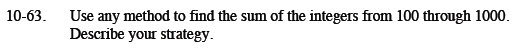Home > CCA2 > Chapter 10 > Lesson 10.1.4 > Problem10-63

10-63.

Use any method to find the sum of the integers from 100 through 1000. Describe your strategy. Homework Help ✎1000 + 100 = 1100
There are 901 integers between 100 and 1000, inclusive.

Substitute these numbers into the formula:

$\frac{901(1000+100)}{2}$

495,550